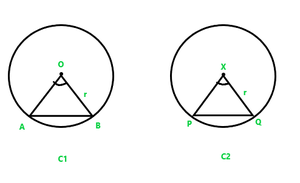# Class 9 NCERT Solutions – Chapter 10 Circles – Exercise 10.2

### Question 1. Recall that two circles are congruent if they have the same radii. Prove that equal chords of congruent circles subtend equal angles at their centres.

Solution:Given:

Two Congruent Circles C1 and C2

AB is the chord of C1

and PQ is the chord of C2

AB = PQ

To Prove: Angle subtended by the Chords AB and PQ are equal i.e. ∠AOB = ∠PXQ

Proof:

In △AOB & △PXQ

AO = PX                    (Radius of congruent circles are equal)

BO = QX                      (Radius of congruent circles are equal)

AB = PQ                    (Given)

△AOB ⩭ △PXQ       (SSS congruence rule)

Therefore, ∠AOB = ∠PXQ         (CPCT)

### Question 2. Prove that if chords of congruent circles subtend equal angles at their centres, then the chords are equal.

Solution:Given:

Two Congruent circles C1 and C2

AB is the chord of C1 and PQ is chord of C2

& ∠AOB = ∠PXQ

To prove :

In △AOB and  △PXQ ,

AO = PX                         (Radius of congruent circles are equal)

∠AOB = ∠PXQ              (Given)

BO = QX                          (Radius of congruent circles are equal)

△AOB ⩭ △PXQ          (SAS congruence rule)

Therefore, AB = PQ       (CPCT)

Whether you're preparing for your first job interview or aiming to upskill in this ever-evolving tech landscape, GeeksforGeeks Courses are your key to success. We provide top-quality content at affordable prices, all geared towards accelerating your growth in a time-bound manner. Join the millions we've already empowered, and we're here to do the same for you. Don't miss out - check it out now!

Previous
Next